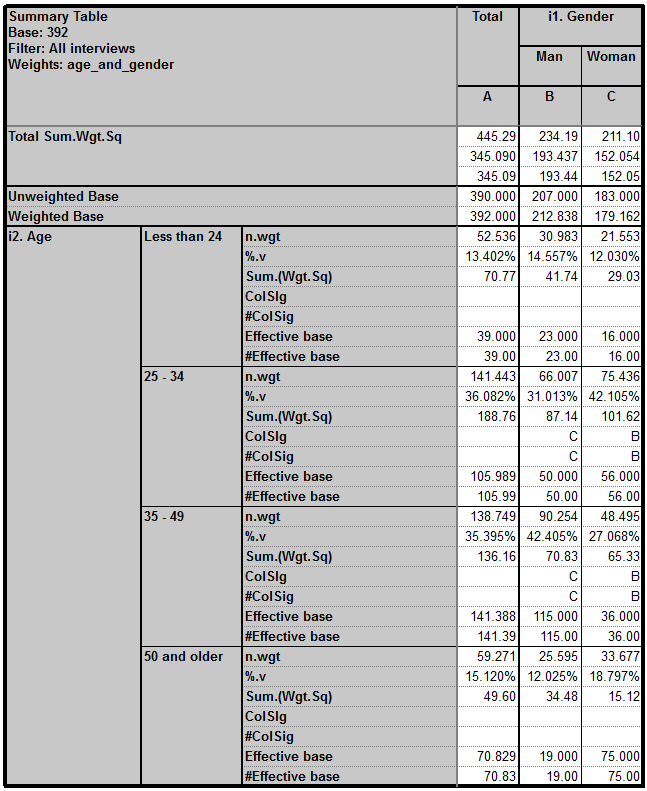Scroll

# Programming Col Sig (proportions): Part 3 - (Student Test using efficiency coefficient)

Follow
 Summary This article demonstrates how to script the formula for Student Test using efficiency coefficient on closed questions. Formula and syntax are shown. There is also a demonstration of calculating Effective base manually. Applies to Askia Analyse. Written for Researchers, Developers, Data Processors. Keywords Table arithmetic, Forloops, Coordinates, data, Calculation arithmetic, Bespoke calculation, Filtered, Multiplication table, Classical Student Test, Sigma2, Sigma3, Z-test, Unpooled variance, Confidence interval, 95%, FilterBy, GetColSigLetter, Effective Base, Weighting, Generate intermediary results, DebugTrace, DebugColSig.

The .qes file and portfolio containing the examples discussed is attached: Table Arithmetic Examples 11 & 12.rar.

Student Test using efficiency coefficient (Sigma3)

In this demonstration I will create a table which shows the pre-set colsig calculation side by side with the programmed calculation for the same test. First, let's review the formula: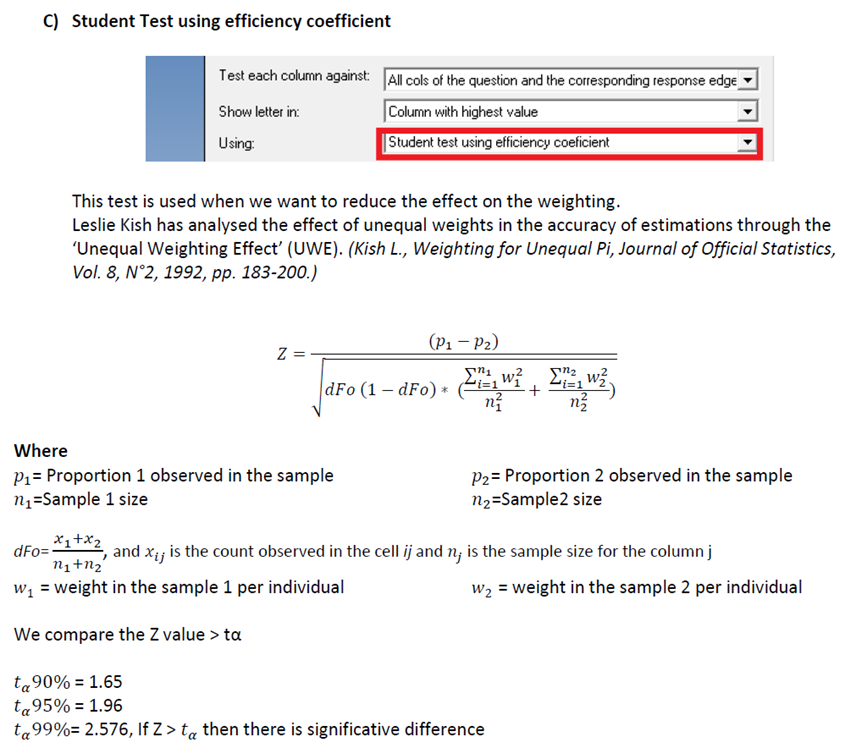Create a variable based on your weighting: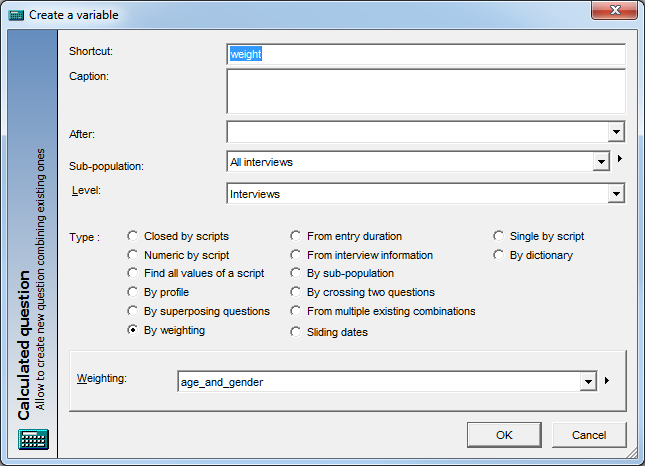Create a second variable ‘weight sq’ which is the square of weighting factors stored in this variable, ‘weight’.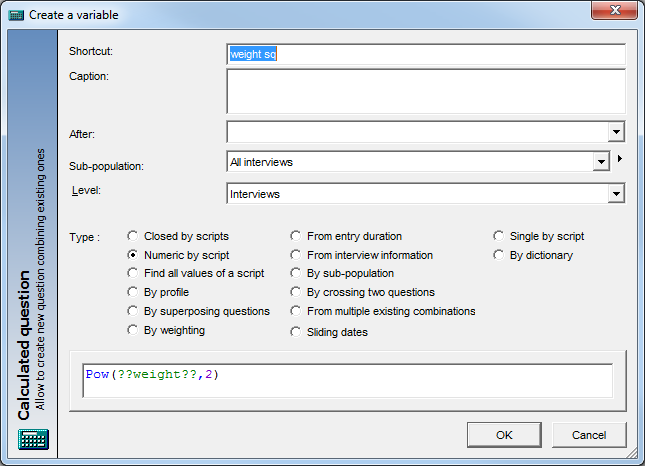Create your table: in this case it is Age by Gender.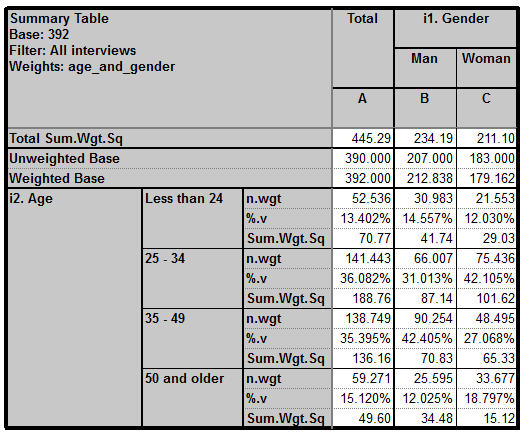Note the calculations applied: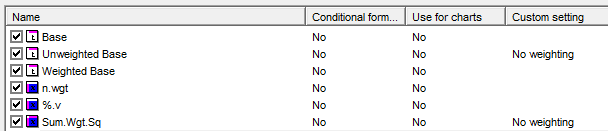Sum.Wgt.Sq is a Script (sum) calculation based on our squared weighting factors: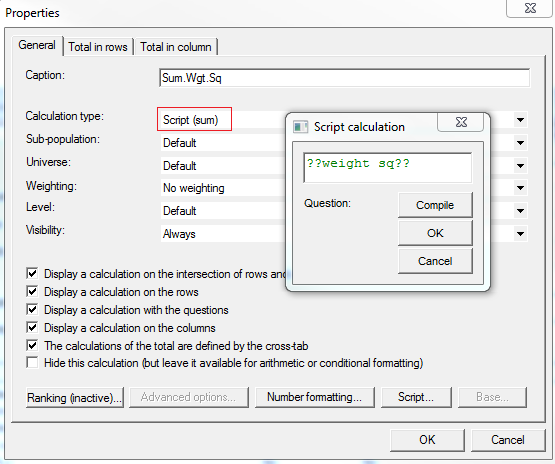Apply the calculation for colsig (in built) and a calculation arithmetic calculation where we will program Student test using efficiency coefficient: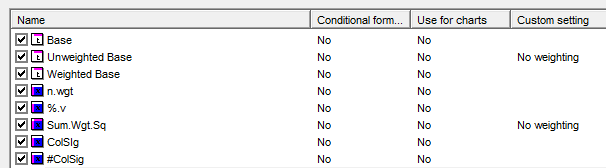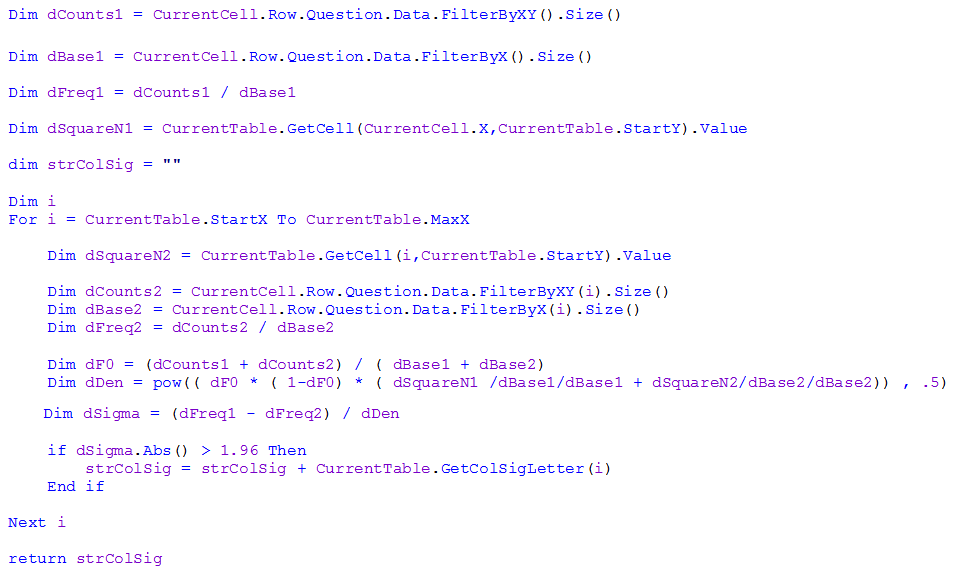Get the result: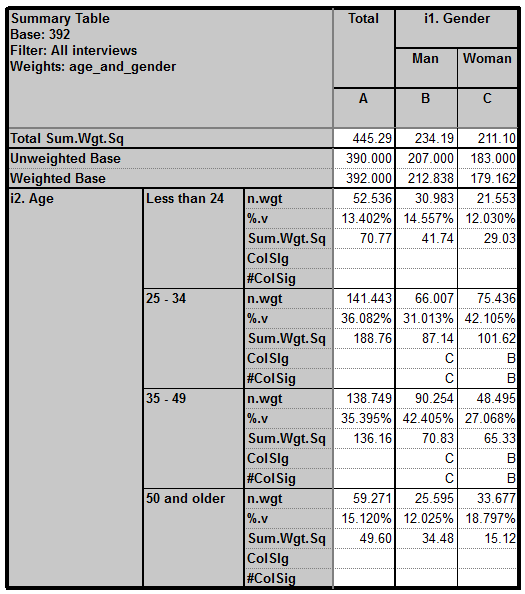You can use debug trace commands in the script to create a helpful file (DebugTrace.txt) in the .dat folder: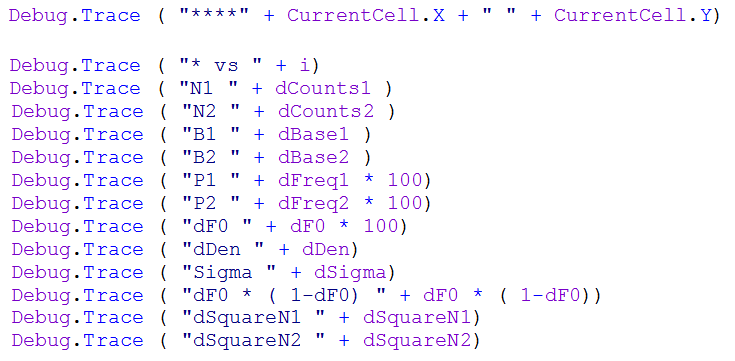This file is included. You can output different values that different parts of the syntax are returning. It is very helpful for working out errors in your syntax.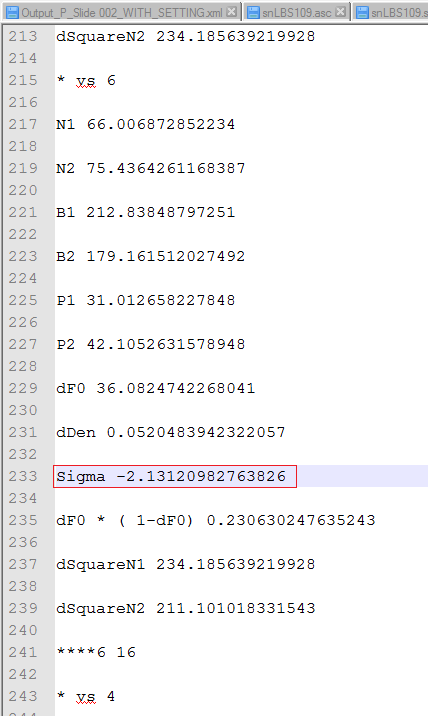You can compare this to the figures output in the (DebugColSig.txt) file also output in the .dat folder (for in-built col sig)*.

* Generate intermediary results when calculating col-sig must be set to 'Yes' in your Analyse options.

In (DebugColSig_S3.xlsx) you can see I have extended this and used manual Excel calculation to calculate Sigma 3.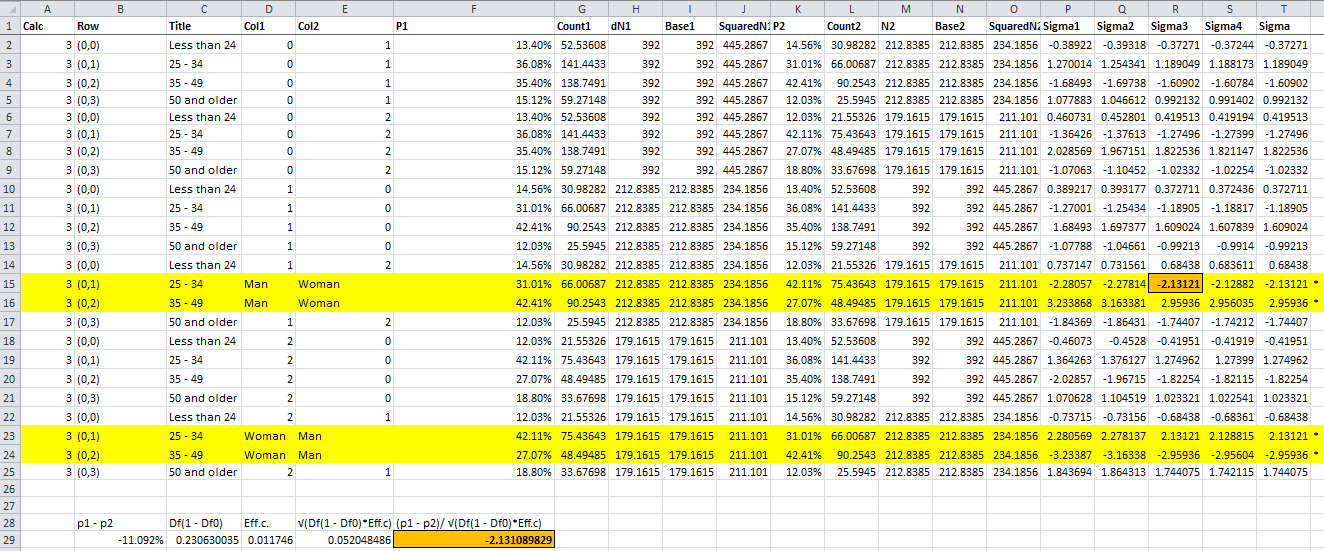In the Sigma3 tab definition you can see that there are additional calculations showing: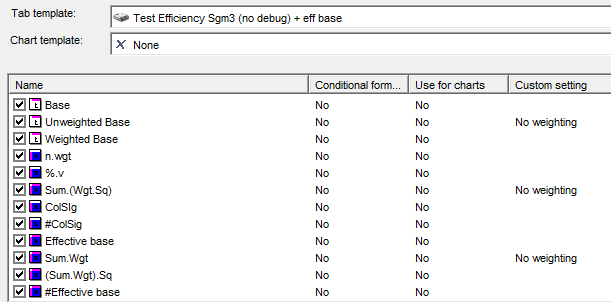Effective base & #Effective base. The latter is the manual calculation. This is the (sum of weight factors) squared, divided by the sum of the squared weight factors.

To achieve this, the key is to have the following variables:

weight  (weighting factors)
weight sq  (weighting factors squared)

Then to have these cross calculations:

Sum.(Wgt.Sq) - Script (sum) - ??weight sq?? - Calc(3) in example file
Sum.Wgt - Script (sum) - ??weight?? - Calc(7) in example file
(Sum.Wgt).Sq - Calculation arithmetic - Calc(7) * Calc(7) - Calc(8) in example file
#Effective base - Calculation arithmetic - Calc(8) / Calc(3)

The result is this: